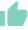# Annualized Return in Mutual FundsGaurav Seth
20 Likes | 754 days agoLikeShareAuthor is a Zfunds Verified ExpertGaurav Seth
Gurugram## Annualized Return in Mutual Funds

Return is the profit or gain that is generated from the investment over a period of time. There are many different metrics that are used to calculate returns and one of them is annualized returns. In this article, we will discuss in detail about the annualized returns and how does it work.

## Annualized Returns

As the name suggests, annualized returns show the annual returns of an investment.

The return generated from an investment over a period of time is known as the absolute return but afterward, to compare the annual returns, absolute returns are converted into annualized returns.

## Formula to calculate annualized returns:

Annualized return =  (((1 +  Absolute return) ^ (12/no. of months)) - 1 ) * 100

Or

Annualized return =  (((1 + Absolute return) ^ (1/no. of years)) - 1 ) * 100

Or

Annualized return =  [((1 + R1) * (1 + R2) * (1 + R3) * ….. * (1 + RN)) ^ (1/N)]  - 1

## How does Annualized return work?

Let us understand it with an example.

Mr. X made an investment of Rs. 1,50,000 done on 1st January 2020 in ABC mutual fund for 1,500 units. All the 1,500 units were redeemed on 31st December 2021. In 2020, Mr. earned a return of 10%, and in 2021, a return of 13%.

Annualized return =  [((1 + R1) * (1 + R2) * (1 + R3) * ….. * (1 + RN)) ^ (1/N)]  - 1

Annualized return =  [((1 + 10%) * (1 + 13%)) ^ (½)] - 1

Annualized return = 11.49%

The annualized return for the investment is 11.49%. It means that Mr. X has earned 11.49% annually on an average on his investment.

## How the Annualized return is different from CAGR?

Let us first understand what is CAGR.

CAGR or Compounded Annual Growth Rate shows the annual return of an investment. It shows the geometric return on investment over the time period.

Similarly, annualized return also shows the annual return of an investment. So, the question is what is the difference between them? There is very little difference between them and many times both returns when calculated come out to be the same. The only difference between them is, CAGR shows the annual return over a period of time or the total investment tenure using the beginning and end value of the investment while annualized returns do calculations by using the returns from several annual years.

Let us understand it with an example.

Mr. X invested Rs. 1,00,000 on 1st January 2016.

Let us assume, he earns the following return over his investment:

Annualized return =  [((1 + R1) * (1 + R2) * (1 + R3) * ….. * (1 + RN)) ^ (1/N)]  - 1

Annualized return =  [((1 + 9%) * (1 + 11%) * (1 + 10%) * (1 + 11%) * (1 + 8%)) ^ (⅕)]  - 1

Annualized return =  9.8%

CAGR = (((Final value /  Beginning value ) ^ (1 / No. of years)) - 1 ) * 100

CAGR = (((1,59,547.10 / 1,00,000) ^ (1 / 5)) - 1 ) * 100

CAGR = 9.8%

As we can see, the returns are the same using both the calculations i.e annualized returns & CAGR returns.

• What is annualized return?

Annualized return is a return metric that shows the annualized return of an investment for a specific time period. It tells how much an investor earns or can expect on his investment annually over the specified period.

• What is the formula to calculate annualized return?

The formula to calculate the annualized returns are :

Annualized return =  (((1 +  Absolute return) ^ (12/no. of months)) - 1 ) * 100

Or

Annualized return =  (((1 + Absolute return) ^ (1/no. of years)) - 1 ) * 100

Or

Annualized return =  (((1 + Absolute return) ^ (52/no. of weeks)) - 1 ) * 100

Or

Annualized return =  (((1 + Absolute return) ^ (365/no. of days )) - 1 ) * 100

Or

Annualized return =  [((1 + R1) * (1 + R2) * (1 + R3) * ….. * (1 + RN)) ^ (1/N)]  - 1

• What does annualized return show?

The annualized return shows the compounded annual rate of return during the period of investment. Like, if an investor invested for 5 years, so to check what an investor would have earned provided the returns compounded annually, he/she can calculate the annualized return.

• What is the difference between the annualized return and CAGR?

Generally, CAGR and annualized returns are almost the same. In fact, these two terms are used interchangeably across investors. There is a very minor difference between them i.e the way in which they are calculated. CAGR shows the annual growth rate of investment during the whole investment tenure by using the beginning & end value of investments. The annualized returns show the annual growth rate for the specified tenure using the annual returns of the several periods.